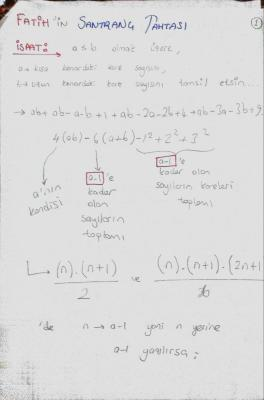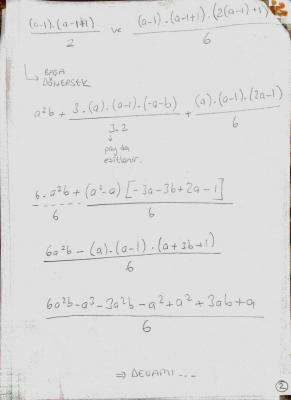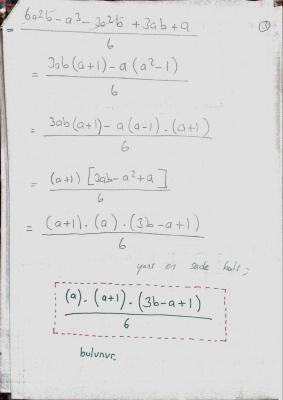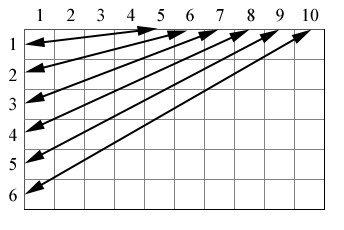# My Math Theorem : Square

## Recommended Posts

Interesting.

##### Share on other sites

Actually this theorem answer this combination question: "how many squares are in The chessboard? " or in The puzzle newspaperthanks For answering)

##### Share on other sites

Maybe I'm not seeing the point. Since you already know a and b, why not just multiply them?

##### Share on other sites

Maybe I'm not seeing the point. Since you already know a and b, why not just multiply them?

Number of small squares AND number of squares made from groups of smaller squares (2x2, 3x3 etc) - I presume this as I have not checked the maths

Fatih

Nice one Fatih. These proofs will get you more credit if you show more workingBut your formula seems to work for me.

I did it to double check your numerical result - but not to check your formula

[mp][/mp]

I did it by first considering your 7*9 example to produce a formula for a 7*9

BTW - this is a quick scribble and unchecked; I hope I havent made a big mistakeI then generalised this equation (note I set b=a+n)I then simplified this equation to give a simple formula - and then checked that it was the same as yours by making the same substitution of b=a+n in your formula##### Share on other sites

@fatih I aprreciate your interest ,but I am smiling:-)

because you have not proved the allegement you have given. this is principle at math departments and also this principle is certified/valid in other disciplines of science.

I will simply express that ;

"it is not proof giving example.":-)

Edited by blue89
##### Share on other sites

Number of small squares AND number of squares made from groups of smaller squares (2x2, 3x3 etc) - I presume this as I have not checked the maths

Ok, that makes more sense. I couldn't quite to what he was trying to do. Thanks!

##### Share on other sites

Thanks For answering.bravo imatfaal !Blue89 okay.I will add my proof.Thanks!

But i have a question.My teacher said "Fatih you're idiot.You don't have mathematicial ability" but i want to be a mathematician so i feel blue.what do you think i should do?

My proof is ;##### Share on other sites

in relevancy of your mathematical allegement ,I implied that this was principle at mathematics ,using determined methods to prove something.

for instance proving by creating contradiction , proving by log.induction , ..etc. but I am quite sure that giving example is not proof.

it is true that mathematics is entertaining science ,but it requires to study hard.

(I think) if someone is willing to learn and believe that he/she has creative and intelligent personality ,I highly recommend that going on studying mathematics.

!!: (note: contradiction with your allegement exists : let give value a=4 ,b=8 ,this formula does not trust giving these values ,and also your calculations seems like including errorrs (incorectness)) you can send them to me in private. I can assess if I will have enough time then)

!!:these can not show that you would be unsuccessfull at mathematics in the future!!..

additional imnformation : I advise that you check K-12 community in american education system.

Edited by blue89
##### Share on other sites

It seems I lost you somewhere. I thought I understood your first post, and I can follow all the mathematics, but what does this find? Does it find the number of squares that can be multiplied to become that number or is it something else? I'm loamerica.

Edited by Raider5678
##### Share on other sites

But i have a question.My teacher said "Fatih you're idiot.You don't have mathematicial ability" but i want to be a mathematician so i feel blue.what do you think i should do?

Congratulations Fatih!!! The equation you derived is absolutely correct, and you definitely have mathematical ability! I truly believe that if you love mathematics, then you should definitely pursue it regardless of what your teacher or anyone else thinks of you (shame on your teacher for saying you're an idiot). I started out doing the same type of mathematics that you have demonstrated here in this thread and it grew into a love for the subject that allowed me to explore and discover new mathematics (new to me anyway). Even if the equations that you discover are already known, the fact that you took the time to figure them out for yourself places you far ahead in the game. Don't let anyone deter you from doing the things you love. As for your equation:

Given $m$ rows and $n$ columns of 1x1 squares where $m \le n$, we can construct $(m-1)$ rows and $(n-1)$ columns of 2x2 squares, $(m-2)$ rows and $(n-2)$ columns of 3x3 squares, and so forth until we reach $(m-m+1)$ rows and $(n-m+1)$ columns of $m$ x $m$ squares.

Using the upper limits and working backwards, we can show that there are $(1)(n-m+1)$ number of $m$ x $m$ squares, $(2)(n-m+2)$ number of $(m-1)$ x $(m-1)$ squares, $(3)(n-m+3)$ number of $(m-2)$ x $(m-2)$ squares, and so forth such that for any $(m-i+1)$ x $(m-i+1)$ sized square where $1 \le i \le m$ there are a total of $(i)(n-m+i)$ squares. To find the total number of squares in the $m$ x $n$ rectangle, all we have to do is sum $(i)(n-m+i)$ on the interval $[1,m]$:

$\sum_{i=1}^m\,i\left(n-m+i\right)=\sum_{i=1}^m\,n\,i-\sum_{i=1}^m\,m\,i+\sum_{i=1}^m\,i^2$

Find the polynomial solutions for each sum:

$\sum_{i=1}^m\,n\,i=\frac{n(m)(m+1)}{2}$

$\sum_{i=1}^m\,m\,i=\frac{m^{2}(m+1)}{2}$

$\sum_{i=1}^m\,i^2=\frac{m(m+1)(2m+1)}{6}$

Simplify the resulting algebra:

$\frac{n(m)(m+1)}{2}-\frac{m^{2}(m+1)}{2}+\frac{m(m+1)(2m+1)}{6}=\frac{m(m+1)(3n-m+1)}{6}$

Proving that the total number of squares in a $m$ x $n$ rectangle of 1x1 squares is defined by the equation:

$\frac{m(m+1)(3n-m+1)}{6}$

The following image demonstrates the process for a $6 \times 10$ rectangle:Number of 6x6 squares equals $1 \times 5 = 5$

Number of 5x5 squares equals $2 \times 6 = 12$

Number of 4x4 squares equals $3 \times 7 = 21$

Number of 3x3 squares equals $4 \times 8 = 32$

Number of 2x2 squares equals $5 \times 9 = 45$

Number of 1x1 squares equals $6 \times 10 = 60$

Total number of squares in a $6 \times 10$ rectangle is equal to

$5+12+21+32+45+60=175$

or

$\frac{6(6+1)(3(10)-6+1)}{6}=175$

When $m=n$ we get back the polynomial for $\sum x^2$ as expected:

$\sum_{i=1}^m\,i^2=\frac{m(m+1)(3m-m+1)}{6}=\frac{m(m+1)(2m+1)}{6}$

Edited by Daedalus
##### Share on other sites

My teacher said "Fatih you're idiot.You don't have mathematicial ability" but i want to be a mathematician so i feel blue.what do you think i should do?

I am not sure I should be saying this on a public forum, but... I failed mathematics at high school.

And I know I am not the only one who did not do so well at school in mathematics who has now gone on to contribute to mathematics. High school is not like mathematics research and creativity is needed rather than just being able to recall the methods quickly. You need to understand why they work and be able to modify the methods.

In short, don't give up quite yet.

*******************

Maybe this could be split off into a new thread?

Edited by ajb
##### Share on other sites

Fatih

If you want a further - and I would guess - higher challenge what about generalising to the number of rectangles in a given rectangular grid

Merged post follows:

[/mp]

....

*******************
Maybe this could be split off into a new thread?

So that you can hide your admission - not a chance. You are lucky we don't pin that post to the top of the forum :-D

[mp]

I have just done the rectangles in a rectangular grid and it is not much harder once you see the patterns and are careful to be neat and consistent in how you count

##### Share on other sites

I am not sure I should be saying this on a public forum, but... I failed mathematics at high school.

And I know I am not the only one who did not do so well at school in mathematics who has now gone on to contribute to mathematics. High school is not like mathematics research and creativity is needed rather than just being able to recall the methods quickly. You need to understand why they work and be able to modify the methods.

In short, don't give up quite yet.

*******************

Maybe this could be split off into a new thread?

I failed Algebra in elementary and high school. I'm currently in a Computer Science program(which requires major knowledge of mathematics), with a 3.5 GPA. As ajb said, don't give up. You have talent. Sometimes different teaching styles are required.

Edited by Unity+
##### Share on other sites

Thank you very much) i will not give up.You made me so happy ! Thanks for all good comments !## Create an account

Register a new account